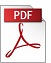### “Maximal imaginery eigenvalues in optimal systems”

Authors: David Di Ruscio,
Affiliation: NTNU, Department of Engineering Cybernetics
Reference: 1991, Vol 12, No 3, pp. 149-158.

Keywords: Linear optimal control, pole placement, eigenvalues, multivariable control systems, control system design

Abstract: In this note we present equations that uniquely determine the maximum possible imaginary value of the closed loop eigenvalues in an LQ-optimal system, irrespective of how the state weight matrix is chosen, provided a real symmetric solution of the algebraic Riccati equation exists. In addition, the corresponding state weight matrix and the solution to the algebraic Riccati equation are derived for a class of linear systems. A fundamental lemma for the existence of a real symmetric solution to the algebraic Riccati equation is derived for this class of linear systems.PDF (1072 Kb)        DOI: 10.4173/mic.1991.3.5

  D. Di Ruscio (1991), doi:10.1109/CDC.1991.261580  D. Di Ruscio (1992), doi:10.1109/CDC.1992.371469
References:
 DI RUSCIO, D. BALCHEN, J.G. (1990). A Schur method for designing LQ-optimal systems with prescribed eigenvalues, Modeling, Identification and Control, 11, 55-72 doi:10.4173/mic.1990.1.5
 KUCERA, V. (1989). Algebraic Riccati equation: Symmetric and definite solutions, Workshop on The Riccati equation in control systems, and signals. Como, Italy 1989. With the participation of IEEE-Control Systems Society. Printed by Pitagora Editrice Bologna, Via del Legatore 3, Bologna.
 LANCASTER, P. RODMAN, L. (1980). Existence and uniqueness theorems for the algebraic Riccati equation, Int. J. Control, 32, 285-309 doi:10.1080/00207178008922858
 LAUB, A.J. (1979). A Schur Method for Solving Algebraic Riccati Equations, IEEE Trans. on Automatic Control, 24, 913-921 doi:10.1109/TAC.1979.1102178
 WILLEMS, J.C. (1971). Least Squares Stationary Optimal Control and the Algebraic Riccati Equation, IEEE Trans. on Automatic Control, 16, 621-634 doi:10.1109/TAC.1971.1099831

BibTeX:
@article{MIC-1991-3-5,
title={{Maximal imaginery eigenvalues in optimal systems}},
author={Di Ruscio, David},
journal={Modeling, Identification and Control},
volume={12},
number={3},
pages={149--158},
year={1991},
doi={10.4173/mic.1991.3.5},
publisher={Norwegian Society of Automatic Control}
};